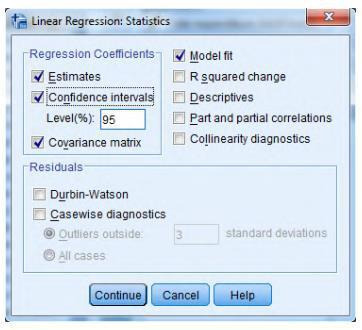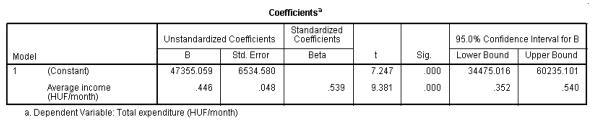# Correlation and Regression Examples Using SPSS Statistics

If you are attending statistics classes or need to make a certain analysis of data for your research, SPSS Statistics software will be an undeniable helper. Learn from the best correlation and regression examples on our blog to have a better understanding of how to use SPSS Statistics. All sample tasks that we post on our blog are supported with illustrations and detailed descriptions, so you can easily understand the process and be able to do everything by yourself.

Read the sample attentively and highlight the key moments. We will walk you through the complete procedure that you will use in SPSS Statistics, even if you have just started using this software. Also, if you are new to SPSS Statistics we recommend you to learn the interface of the program and basic functions to easily navigate in the program. To find more completed tasks and correlation and regression examples, check out the fist part of this regression statistics example and visit our blog.

You can also send us do my statistics homework free request when you need qualified help and get it any time .

## Linear Regression is SPSS Statistics. Part 2

Task: take a closer look at how to do correlation and linear regression is SPSS Statistics (Continuation).

Example 1

Determine 95% confidence interval for the b1 parameter.

For displaying the confidence interval of b coefficients, click on the box next to ‘Confidence intervals’ and change the confidence level, if it is necessary.The following figure contains the confidence interval for b coefficient at 95% confidence level.The b1 coefficient is between 0.352 and 0.540 at a 95% confidence level.

Example 2

Determine 95% confidence interval for the dependent variable.

To display the upper and lower bounds for mean or individual prediction interval, click on the box of ‘Mean’ or ‘Individual.’ Enter 95% confidence interval.

Therefore, the current salary of employees is between 54 938.72 and 225 794.08 at a

95% confidence level.

If you need help with assignment, you can contact our specialists 24/7 and tell them your problem.